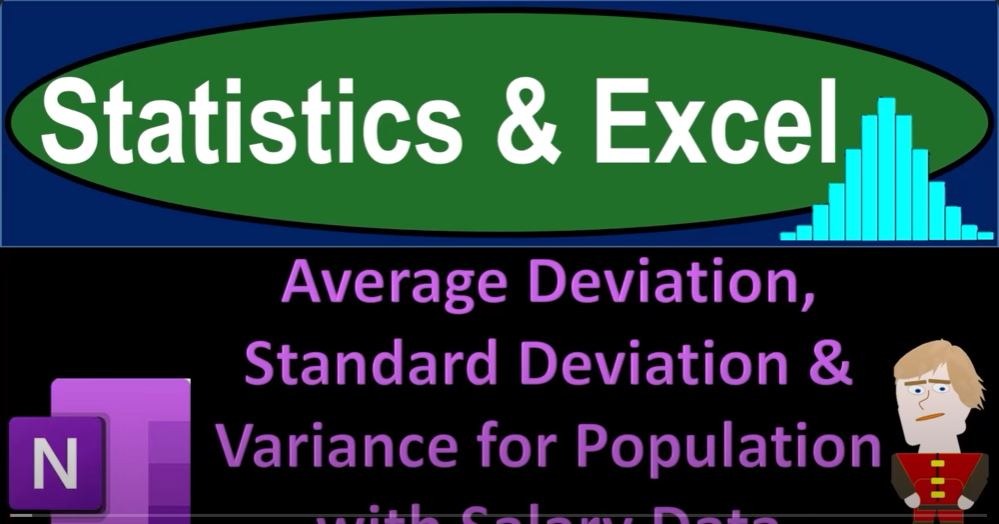## Average Deviation, Standard Deviation & Variance for Population with Salary Data 1443

In the world of data analysis and statistics, the understanding of key concepts such as average deviation, standard deviation, and variance is crucial. These metrics provide insights into the dispersion and distribution of data. In this blog, we will delve into these statistical concepts using Excel, focusing on a population of salary data as an example.

Before we dive into the calculations, we’d like to mention that we will be using Microsoft OneNote and Excel, making it easier for you to follow along. We’ll provide a step-by-step guide on how to perform these calculations and then demonstrate the results visually using OneNote.

Average (Mean)

The mean, or average, is a fundamental statistic that represents the center point of a dataset. To calculate the average in Excel, you can simply use the AVERAGE function. For our salary data, we found the mean to be \$71,498.

Minimum and Maximum

The minimum and maximum values in your dataset are the lowest and highest data points, respectively. Excel’s MIN and MAX functions make these calculations easy. In our example, the minimum salary is \$12,509, and the maximum is \$84,000.

Quartiles

Quartiles are values that divide your dataset into four equal parts. Excel’s QUARTILE function can help you find these values. For quartile one, we obtained \$69,800, and for quartile three, we found \$74,700. The median, also known as quartile two, is \$71,498, the same as the mean.

Average Deviation

To understand how each data point deviates from the mean, you can calculate the average deviation. This involves finding the absolute difference between each data point and the mean and then averaging these differences. Although this method provides valuable insights into individual data points, it doesn’t help us analyze the overall spread of data.

Variance

Variance is a more advanced statistical concept. It represents the average of the squared differences between each data point and the mean. The formula for variance is Sigma², and in our example, the population variance is approximately 75,676.43.

Standard Deviation

Standard deviation is closely related to variance. It is the square root of the variance and provides a measure of how data points deviate from the mean. In our example, the standard deviation for the population is approximately \$2,750.

Excel Functions for Variance and Standard Deviation

Excel provides built-in functions to calculate variance and standard deviation. You can use VAR.P (population variance) and STDEV.P (population standard deviation) functions. These functions can make your calculations more efficient, especially when dealing with larger datasets.

Conclusion

Understanding and using statistics, such as average deviation, standard deviation, and variance, is essential for anyone working with data. These metrics allow us to gain insights into the distribution and spread of data points. In Excel, you can easily perform these calculations using built-in functions.

Keep in mind that while some of these concepts may seem abstract, they become more meaningful when comparing different datasets or drawing conclusions about the variability of data. In future presentations, we will explore the practical applications of standard deviation and variance in more depth. Excel’s versatility and ease of use make it a valuable tool for data analysis, and mastering these statistical concepts will enhance your analytical skills.